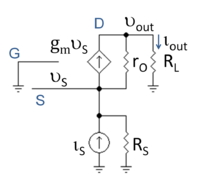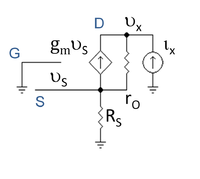# Common gate

Common gateFigure 1: Basic N-channel common-gate circuit (neglecting biasing details); current source ID represents an active load; signal is applied at node Vin and output is taken from node Vout; output can be current or voltage

In electronics, a common-gate amplifier is one of three basic single-stage field-effect transistor (FET) amplifier topologies, typically used as a current buffer or voltage amplifier. In this circuit the source terminal of the transistor serves as the input, the drain is the output and the gate is common to both, hence its name. The analogous bipolar junction transistor circuit is the common-base amplifier.

## Applications

This configuration is used less often than the common source or source follower. It is useful in, for example, CMOS RF receivers, especially when operating near the frequency limitations of the FETs; it is desirable because of the ease of impedance matching and potentially has lower noise. Gray and Meyer provide a general reference for this circuit.

## Low-frequency characteristicsFigure 2: Small-signal low-frequency hybrid-pi model for amplifier driven by a Norton signal source

At low frequencies and under small-signal conditions, the circuit in Figure 1 can be represented by that in Figure 2, where the hybrid-pi model for the MOSFET has been employed.Figure 3: Hybrid pi model with test source ix at output to find output resistance

The amplifier characteristics are summarized below in Table 1. The approximate expressions use the assumptions (usually accurate) rO >> RL and gmrO >> 1.

Table 1 Definition Expression Approximate expression
Short-circuit current gain${A_{i}} = {i_\mathrm{out} \over i_\mathrm{S}} \Big|_{R_{L}=0}$$\ 1$$\ 1$
Open-circuit voltage gain${A_{v}} = {v_\mathrm{out} \over v_\mathrm{S}} \Big|_{R_{L}=\infty}$$\begin{matrix} (g_m r_\mathrm{O}+1) \frac {R_L} {r_O + R_L} \end{matrix}$$\ g_m R_L$
Input resistance$R_\mathrm{in} = \frac{v_{S}}{i_{S}}$${{R_L+r_O} \over {g_m r_O +1}}$$\begin{matrix} \frac {1} {g_m} \end{matrix}$
Output resistance$R_\mathrm{out} =\frac{v_{x}}{i_{x}}$$\ (1+g_m r_O)R_S + r_O$ rO
Note: Parallel lines (||) indicate components in parallel.

In general the overall voltage/current gain may be substantially less than the open/short circuit gains listed above (depending on the source and load resistances) due to the loading effect.

### Closed circuit voltage gain

Taking input and output loading into consideration, the closed circuit voltage gain (that is, the gain with load RL and source with resistance RS both attached) of the common gate can be written as:${A_\mathrm{v}} \approx \begin{matrix} \frac {g_m R_\mathrm{L}} {1+g_mR_S} \end{matrix}$ ,

which has the simple limiting forms$A_\mathrm{v} = \begin{matrix} \frac {R_L}{R_S}\end{matrix} \ \ \mathrm{ or } \ \ A_\mathrm{v} = g_m R_L$,

depending upon whether gmRS is much larger or much smaller than one.

In the first case the circuit acts as a current follower, as understood as follows: for RS >> 1/gm the voltage source can be replaced by its Norton equivalent with Norton current vThév / RS and parallel Norton resistance RS. Because the amplifier input resistance is small, the driver delivers by current division a current vThév / RS to the amplifier. The current gain is unity, so the same current is delivered to the output load RL, producing by Ohm's law an output voltage vout = vThévRL / RS, that is, the first form of the voltage gain above.

In the second case RS << 1/gm and the Thévenin representation of the source is useful, producing the second form for the gain, typical of voltage amplifiers.

Because the input impedance of the common-gate amplifier is very low, the cascode amplifier often is used instead. The cascode places a common-source amplifier between the voltage driver and the common-gate circuit to permit voltage amplification using a driver with RS >> 1/gm.

Wikimedia Foundation. 2010.

### Look at other dictionaries:

• Common source — Figure 1: Basic N channel JFET common source circuit (neglecting biasing details). Figure 2: Basic N channel JFET common source circuit with source degeneration …   Wikipedia

• Common emitter — Figure 1: Basic NPN common emitter circuit (neglecting biasing details). In electronics, a common emitter amplifier is one of three basic single stage bipolar junction transistor (BJT) amplifier topologies, typically used as a voltage amplifier.… …   Wikipedia

• Common base — Figure 1: Basic NPN common base circuit (neglecting biasing details). In electronics, a common base (also known as grounded base) amplifier is one of three basic single stage bipolar junction transistor (BJT) amplifier topologies, typically used… …   Wikipedia

• Common collector — Figure 1: Basic NPN common collector circuit (neglecting biasing details). In electronics, a common collector amplifier (also known as an emitter follower or BJT voltage follower) is one of three basic single stage bipolar junction transistor… …   Wikipedia

• Common drain — In electronics, a common drain amplifier, also known as a source follower, is one of three basic single stage field effect transistor (FET) amplifier topologies, typically used as a voltage buffer. In this circuit the gate terminal of the… …   Wikipedia

• Common Tunnel — Grønland Station Overview Type Rapid transit …   Wikipedia

• Gate House — is one of the four Upper Houses of the Burwash Hall residence at Victoria College in the University of Toronto. Until 2007, when Victoria administration made it co ed, Gate House was the last remaining all male residence building in the… …   Wikipedia

• Common Murre — Uria aalge aalge in breeding plumage at Runde (Norway). Note bridled bird in centre. Conservation status …   Wikipedia

• Common Darter — Conservation status Least Concern ( …   Wikipedia

• Gate Keepers — Infobox animanga/Header name = Gate Keepers caption = The Playstation Gate Keepers A.E.G.I.S. cast ja name = ゲートキーパーズ ja name trans = genre = Adventure, Comedy, Fantasy, Science FictionInfobox animanga/Manga title = author = Keiji Gotoh (art)… …   Wikipedia